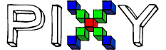# Pixy2 Manual Select Vector

Hello!

I’m working on using the Pixy2’s line tracking function and would like to command the camera to manually select a different line to track when a barcode appears in the viewport. A snippet of the Arduino code I’ve written so far is:

else if (pixy.line.barcodes->m_code==0){
F=pixy.line.numVectors;
Serial.println(“change vector!”);
pixy.line.setMode(LINE_MODE_MANUAL_SELECT_VECTOR);
pixy.line.setVector(F);

``````}
``````

In my mind, this code should cause the detection of the “zero” barcode to trigger a change in which of the two lines in the viewport is the vector, causing the line that is currently green to light up red and the red line to turn some other color. The barcode detection is working fine as evidenced by the print statement in the third line executing successfully. However, the vector does not change from the original one. I’m not sure if I’m running the setVector command properly though, and am wondering if someone can point me in the right direction?Hello,
I believe setVector takes the index of the line. You can find the index by looking at the array of Vectors.

https://docs.pixycam.com/wiki/doku.php?id=wiki:v2:line_api

For example:

``````for (i=0; i<pixy.line.numVectors; i++)
{
if (some condition of pixy.line.vectors[i])
{
index = pixy.line.vectors[i].m_index;
pixy.line.setVector(index);
}
}
``````

Hope this helps

Edward

Hi Edward,

For the bit of code you sent, what is the difference between the counting variable i and the index attribute m_index? This seems to be drilling down to the problem, as the two do not seem to be the same as evidenced by the following code:

The following short block of code gives a serial output of
0
1

``````for (i=0; i<=pixy.line.numVectors; i++)
{
Serial.println(i);
}
``````

As opposed to this block of code, which gives a serial output of
0
0

``````for (i=0; i<=pixy.line.numVectors; i++)
{
Serial.println(pixy.line.vectors[i].m_index);
}
``````

With two vectors that are visible in the viewport, it seems like both of them have m_index values of 0, so I’m not sure how to select one versus the other to use in the setVector command.

Hmm, that’s odd. They numbers should be different. Are you calling getAllFeatures() before you look into the vectors array?

Edward

That seems to have worked, thanks for the tip!

The only issue I’m having now is that the camera has to lose sight of the line that it is actively tracking as the “vector” first so that there is no red labelled vector shown in Pixymon at all. Then when the barcode is detected, it sets the newest line as the “vector” and labels it. Here’s my code:

``````else if (pixy.line.barcodes->m_code==0){
newestVectorCount=pixy.line.numVectors-1; // indicies of vectors count from zero, not 1, so the "-1" term is needed to properly index